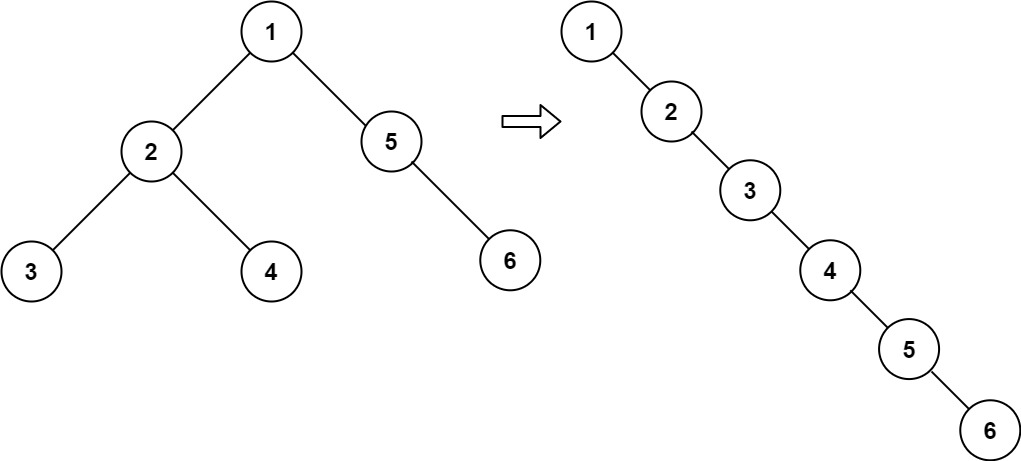## Count Sub Islands LeetCode Solution

Problem Statement Count Sub Islands LeetCode Solution says that grid1 and grid2 contain only 0‘s (representing water) and 1‘s (representing land). The island means the group of 1’s connected 4 directionally. An island in grid2 is considered a sub-island if there is an island in grid1 that contains all the cells that make …

## Vertical Order Traversal of Binary Tree LeetCode Solution

Problem Statement Vertical Order Traversal of Binary Tree LeetCode Solution says – Given the root of a binary tree, calculate the vertical order traversal of the binary tree. For each node at position (row, col), its left and right children will be at positions (row + 1, col – 1) and (row + 1, col + 1) respectively. …

## Sum Root to Leaf Numbers LeetCode Solution

Problem Statement Sum Root to Leaf Numbers LeetCode Solution says – You are given the root of a binary tree containing digits from 0 to 9 only. Each root-to-leaf path in the tree represents a number. For example, the root-to-leaf path 1 -> 2 -> 3 represents the number 123. Return the total sum of all root-to-leaf numbers. Test …

## Binary Tree Inorder Traversal LeetCode Solution

Problem Statement: Binary Tree Inorder Traversal LeetCode solution Given the root of a binary tree, return the inorder traversal of its nodes’ values. Example 1: Input: root = [1,null,2,3] Output: [1,3,2] Example 2: Input: root = [] Output: [] Example 3: Input: root =  Output:  Constraints: The number of nodes in …

## Is Graph Bipartite? LeetCode Solution

Problem Statement Is Graph Bipartite LeetCode Solution- There is an undirected graph with n nodes, where each node is numbered between 0 and n – 1. You are given a 2D array graph, where graph[u] is an array of nodes that node u is adjacent to. More formally, for each v in graph[u], there is an undirected edge between node u and node v. The graph has …

## Design Add and Search Words Data Structure LeetCode Solution

Problem Statement: Design Add and Search Words Data Structure LeetCode Solution says – Design a data structure that supports adding new words and finding if a string matches any previously added string. Implement the WordDictionary class: WordDictionary() Initializes the object. void addWord(word) Adds word to the data structure, it can be matched later. bool search(word) Returns true if there …

## Flatten Binary Tree to Linked List LeetCode Solution

Flatten Binary Tree to Linked List LeetCode Solution says that – Given the `root` of a binary tree, flatten the tree into a “linked list”:

• The “linked list” should use the same `TreeNode` class where the `right` child pointer points to the next node in the list and the `left` child pointer is always `null`.
• The “linked list” should be in the same order as a pre-order traversal of the binary tree.

Example 1:Input:

``` root = [1,2,5,3,4,null,6]
```

Output:

``` [1,null,2,null,3,null,4,null,5,null,6]
```

Example 2:

Input:

``` root = []
```

Output:

``` []
```

Example 3:

Input:

``` root = 
```

Output:

` `

ALGORITHM –

IDEA –

• In order to flatten a binary tree, we will first find the rightmost element of the left subtree and after we got the rightmost element we will link the right-pointer of that node with a right subtree of a given tree.
• In step 2 we will link the right pointer of the root node with the left-subtree and set the left-subtree as null.
• In step 3 now our root node is a right-subtree node same process will happen with this node and the process will still continue until all the left parts become null.

Approach for Flatten Binary Tree to Linked List Leetcode Solution –

– At first, i will run a loop i.e. while(root != null) then will take two variables and store the left-subtree.

– then will check check for the rightmost node of left-subtree by using while(k.left != null) and will link that node with right subtree using (k.right = root.right).

– then link  right pointer of root node with left subtree(root.right = left) and set left pointer of root node as null(root.left=null) and will update by ( root = root.right ) so now root is right subtree node.

– this process will continue until all left-subtree parts become right subtree. Hence, the binary tree will get flattened.## Python Solution:

```class Solution:
def flatten(self, root: Optional[TreeNode]) -> None:
while(root):

if root.left:

k = root.left
temp = root.left

while(k.right):
k = k.right

k.right = root.right

root.right = temp

root.left = None

root = root.right```

## Java Solution:

```class Solution {
public void flatten(TreeNode root) {
while (root != null) {
if (root.left != null) {
TreeNode k = root.left;
TreeNode temp = root.left;
while (k.right != null) k = k.right;
k.right = root.right;
root.right = temp;
root.left = null;
}
root = root.right;
}
}
}```

Time complexity: O(N)

Space Complexity: O(1)

As we have traversed only once, time complexity will be o(n).

and as we haven’t taken any extra space, space complexity will be o(1) constant extra space.

## Diameter of N-Ary Tree LeetCode Solution

Problem Statement : The Diameter of N-Ary Tree LeetCode Solution – Given a root of an N-ary tree, you need to compute the length of the diameter of the tree. The diameter of an N-ary tree is the length of the longest path between any two nodes in the tree. This path may or may not …

## Lowest Common Ancestor of a Binary Tree Leetcode Solution

Problem Statement The Lowest Common Ancestor of a Binary Tree LeetCode Solution – “Lowest Common Ancestor of a Binary Tree” states that given the root of the binary tree and two nodes of the tree. We need to find the lowest common ancestor of these two nodes. The Lowest Common …

## Populating Next Right Pointers in Each Node Leetcode Solution

Problem Statement The Populating Next Right Pointers in Each Node LeetCode Solution – “Populating Next Right Pointers in Each Node” states that given the root of the perfect binary tree and we need to populate each next pointer of the node to its next right node. If there is no next …

Translate »